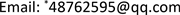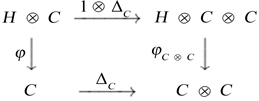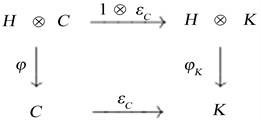﻿ Smash积与Smash余积的对偶性 Duality between the Smash Product and Smash Coproduct

Advances in Applied Mathematics
Vol.06 No.09(2017), Article ID:23091,10 pages
10.12677/AAM.2017.69134

Duality between the Smash Product and Smash Coproduct

Beishang Ren1,2, Yuqiu Wei3, Fenfang Xie2*, Shuai Jin1, Juan Chen1

1Guangdong University of Science & Technology, Dongguan Guangdong

2Guangxi Teachers Education University, Nanning Guangxi

3Guangxi University of Foreign Languages, Nanning GuangxiReceived: Nov. 25th, 2017; accepted: Dec. 14th, 2017; published: Dec. 21st, 2017ABSTRACT

This paper mainly discusses the relations between module algebra and module coalgebra, comodule algebra and comodule coalgebra. Last, from the comodule coalgebra ${A}^{\square }$ contained in ${A}^{0}$ , we further characterize duality between the smash product and smash coproduct.

Keywords:Smash Product, Module Coalgebra, Comodule Coalgebra, Smash CoproduleSmash积与Smash余积的对偶性

1广东科技学院，广东 东莞

2广西师范学院，广西 南宁

3广西外国语学院，广西 南宁Copyright © 2017 by authors and Hans Publishers Inc.1. 引言

(MA1) $A$ 是左 $H$ -模，结构映射 $\gamma :H\otimes A\to A$ ，其中 $\gamma \left(h\otimes a\right)=h\cdot a$

(MA2) $h\cdot \left(ab\right)=\sum \left({h}_{\left(1\right)}\cdot a\right)\left({h}_{\left(2\right)}\cdot b\right),\text{\hspace{0.17em}}\forall h\in H,\text{\hspace{0.17em}}\forall a,b\in A$

(MA3) $h\cdot {1}_{A}={\epsilon }_{H}\left(h\right){1}_{A},\forall h\in H$

(CA1) $A$ 是右 $H$ -余模，结构映射 $\rho :A\to A\otimes H$ ，其中 $\rho \left(a\right)=\sum {a}_{\left(0\right)}\otimes {a}_{\left(1\right)}$

(CA2) $\sum {\left(ab\right)}_{\left(0\right)}\otimes {\left(ab\right)}_{\left(1\right)}=\sum {a}_{\left(0\right)}{b}_{\left(0\right)}\otimes {a}_{\left(1\right)}{b}_{\left(1\right)},\text{\hspace{0.17em}}\forall a,b\in A$

(CA3) $\rho \left(1\right)={1}_{A}\otimes {1}_{H}$

(MC1) $C$ 是左 $H$ -模，结构映射 $\phi :H\otimes C\to C$ ，其中 $\phi \left(h\otimes c\right)\triangleq h\cdot c$

(MC2) ${\Delta }_{C}\left(h\cdot c\right)=\sum {h}_{\left(1\right)}\cdot {c}_{\left(1\right)}\otimes {h}_{\left(2\right)}\cdot {c}_{\left(2\right)},\text{\hspace{0.17em}}\forall h\in H,\text{\hspace{0.17em}}\forall c\in C$

(MC3) $\epsilon \left(h\cdot c\right)={\epsilon }_{H}\left(h\right){\epsilon }_{C}\left(c\right),\text{\hspace{0.17em}}\forall h\in H,\text{\hspace{0.17em}}\forall c\in C$

2. 预备知识

(1) $C$ 是一个左 $H$ -模余代数；

(2) $\phi$ 是余代数同态；

(3) $C$ 的余乘 $\Delta :C\to C\otimes C$ 和余单位 $\epsilon :C\to K$ 都是 $H$ -模同态。

${\Delta }_{C}\phi =\left(\phi \otimes \phi \right)\left(1\otimes T\otimes 1\right)\left({\Delta }_{H}\otimes {\Delta }_{C}\right)$ (2.1)

${\epsilon }_{C}\phi =l\left({\epsilon }_{H}\otimes {\epsilon }_{C}\right)$ (2.2)

${\phi }_{C\otimes C}:H\otimes C\otimes C\to C\otimes C,\text{\hspace{0.17em}}{\phi }_{C\otimes C}=\left(\phi \otimes \phi \right)\left(1\otimes T\otimes 1\right)\left({\Delta }_{H}\otimes 1\otimes 1\right)$

${\phi }_{K}:H\otimes K\to K,\text{\hspace{0.17em}}{\phi }_{K}=l\left({\epsilon }_{H}\otimes 1\right)$

${\Delta }_{C}\phi =\left(\phi \otimes \phi \right)\left(1\otimes T\otimes 1\right)\left({\Delta }_{H}\otimes 1\otimes 1\right)\left(1\otimes {\Delta }_{C}\right)$ (2.3)

${\epsilon }_{C}\phi =l\left({\epsilon }_{H}\otimes 1\right)\left(1\otimes {\epsilon }_{C}\right)$ (2.4)Figure 1. By(2.3) corresponding commutative arrow graphFigure 2. By(2.4) corresponding commutative arrow graph

$\left(M,\phi \right)$ 为左 $H$ -模余代数，则易知 $M$ 必是 ${H}^{\prime }$ -模，其中模结构映射为

${\phi }^{\prime }=\phi \left(f\otimes 1\right)$ ，即 ${h}^{\prime }\circ m=f\left({h}^{\prime }\right)\cdot m,\text{\hspace{0.17em}}\forall {h}^{\prime }\in {H}^{\prime },\text{\hspace{0.17em}}m\in M$ 。于是(MC1)成立。

${\Delta }_{M}\left({h}^{\prime }\circ m\right)=\sum {{h}^{\prime }}_{\left(1\right)}\circ {m}_{\left(1\right)}\otimes {{h}^{\prime }}_{\left(1\right)}\circ {m}_{\left(2\right)}$ 。所以(MC2)成立。

$〈{m}^{\ast }←a,m〉=〈{m}^{\ast },m\cdot a〉,\text{\hspace{0.17em}}\forall m\in M,\text{\hspace{0.17em}}{m}^{\ast }\in {M}^{\ast },\text{\hspace{0.17em}}a\in A$

${C}^{\ast }$ 必然具有代数结构不同的是， $K$ -代数 $A$ 的对偶空间 ${A}^{\ast }$ 未必是余代数。但含在 ${A}^{\ast }$ 内的 ${A}^{\circ }$ 却具

(1) $A$ 是一个左 $H$ -模代数，那么 ${A}^{\circ }$ 是一个右 $H$ -模余代数；

(2) $C$ 是一个左 $H$ -模余代数，那么 ${C}^{\ast }$ 是一个右 $H$ -模代数。

$\begin{array}{l}\forall a,b\in A,〈{M}^{\ast }\left({a}^{\circ }←h\right),a\otimes b〉=〈{a}^{\circ }←h,ab〉=〈{a}^{\circ },h\cdot ab〉\\ =\sum 〈{a}^{\circ },\left({h}_{\left(1\right)}\cdot a\right)\left({h}_{\left(2\right)}\cdot b\right)〉=\sum 〈{M}^{\ast }\left({a}^{\circ }\right),\left({h}_{\left(1\right)}\cdot a\right)\otimes \left({h}_{\left(2\right)}\cdot b\right)〉\\ =\sum 〈{a}_{\left(1\right)}^{\circ }\otimes {a}_{\left(2\right)}^{\circ },\left({h}_{\left(1\right)}\cdot a\right)\otimes \left({h}_{\left(2\right)}\cdot b\right)〉=\sum 〈{a}_{\left(1\right)}^{\circ },{h}_{\left(1\right)}\cdot a〉〈{a}_{\left(2\right)}^{\circ },{h}_{\left(2\right)}\cdot b〉\\ =\sum 〈{a}_{\left(1\right)}^{\circ }←{h}_{\left(1\right)},a〉〈{a}_{\left(2\right)}^{\circ }←{h}_{\left(2\right)},b〉=〈\sum \left({a}_{\left(1\right)}^{\circ }←{h}_{\left(1\right)}\right)\otimes \left({a}_{\left(2\right)}^{\circ }←{h}_{\left(2\right)}\right),a\otimes b〉\end{array}$

(2) 因为 $\left(C,\Delta ,\epsilon \right)$ 是左 $H$ -模余代数，那么自然知 $\left({C}^{\ast },{\Delta }^{\ast },{\epsilon }^{\ast }\right)$ 为代数且 ${C}^{\ast }$ 为右 $H$ -模，所以(MA1)成立。

$\begin{array}{c}〈{c}^{\ast }{d}^{\ast }←h,c〉=〈{\Delta }^{\ast }\left({c}^{\ast }\otimes {d}^{\ast }\right),h\cdot c〉=〈{c}^{\ast }\otimes {d}^{\ast },\Delta \left(h\cdot c\right)〉\\ =\sum 〈{c}^{\ast }\otimes {d}^{\ast },{h}_{\left(1\right)}{c}_{\left(1\right)}\otimes {h}_{\left(2\right)}{c}_{\left(2\right)}〉\end{array}$

$\begin{array}{c}=\sum 〈{c}^{\ast },{h}_{\left(1\right)}\cdot {c}_{\left(1\right)}〉〈{d}^{\ast },{h}_{\left(2\right)}\cdot {c}_{\left(2\right)}〉\\ =\sum 〈{c}^{\ast }←{h}_{\left(1\right)},{c}_{\left(1\right)}〉〈{d}^{\ast }←{h}_{\left(2\right)},{c}_{\left(2\right)}〉\\ =\sum 〈\left({c}^{\ast }←{h}_{\left(1\right)}\right)\otimes \left({d}^{\ast }←{h}_{\left(2\right)}\right),{c}_{\left(1\right)}\otimes {c}_{\left(2\right)}〉\end{array}$

$\begin{array}{l}=\sum 〈\left({c}^{\ast }←{h}_{\left(1\right)}\right)\otimes \left({d}^{\ast }←{h}_{\left(2\right)}\right),\Delta \left(c\right)〉\\ =〈\sum \left({c}^{\ast }←{h}_{\left(1\right)}\right)\left({d}^{\ast }←{h}_{\left(2\right)}\right),c〉\end{array}$

$〈{1}_{{c}^{\ast }}←h,c〉=〈\epsilon ,h\cdot c〉=\epsilon \left(h\cdot c\right)=\epsilon \left(h\right)\epsilon \left(c\right)=〈\epsilon \left(h\right)\epsilon ,c〉=〈\epsilon \left(h\right){1}_{{c}^{\ast }},c〉$

(CC1) $C$ 是左 $H$ -余模，结构映射 $\rho :C\to H\otimes C$ ，其中 $\rho \left(c\right)=\sum {c}_{\left(-1\right)}\otimes {c}_{\left(0\right)}$

(CC2) $\sum {\left({c}_{\left(1\right)}\right)}_{\left(-1\right)}{\left({c}_{\left(2\right)}\right)}_{\left(-1\right)}\otimes {\left({c}_{\left(1\right)}\right)}_{\left(0\right)}\otimes {\left({c}_{\left(2\right)}\right)}_{\left(0\right)}=\sum {c}_{\left(-1\right)}\otimes {\left({c}_{\left(0\right)}\right)}_{\left(1\right)}\otimes {\left({c}_{\left(0\right)}\right)}_{\left(2\right)}$

(CC3) $\sum {c}_{\left(-1\right)}\epsilon \left({c}_{\left(0\right)}\right)=\epsilon \left(c\right){1}_{H},\text{\hspace{0.17em}}\forall c\in C$

$H$ -余模余代数的刻画如下：

$\left({M}_{H}\otimes 1\otimes 1\right)\left(1\otimes T\otimes 1\right)\left(\rho \otimes \rho \right){\Delta }_{C}=\left(1\otimes {\Delta }_{C}\right){\rho }_{C}$ (2.5)

$\left(1\otimes {\epsilon }_{C}\right){\rho }_{C}=\left({u}_{H}\otimes 1\right)l{\epsilon }_{C}$ (2.6)

${\rho }_{C\otimes C}=\left({M}_{H}\otimes 1\otimes 1\right)\left(1\otimes T\otimes 1\right)\left({\rho }_{c}\otimes {\rho }_{c}\right),\text{\hspace{0.17em}}{\rho }_{K}=\left({u}_{H}\otimes 1\right)l$

$\Delta :C\to C\otimes C$ 构成左 $H$ -余模同态当且仅当 ${\rho }_{C\otimes C}{\Delta }_{C}=\left(1\otimes {\Delta }_{C}\right){\rho }_{C}$ ，即(2.5)成立； $\epsilon :C\to K$ 构成左 $H$ -余模同态当且仅当 $\left(1\otimes {\epsilon }_{C}\right){\rho }_{C}={\rho }_{K}{\epsilon }_{C}$ ，即(2.6)成立。 $\square$

$H$ -模代数的讨论中我们曾引入了Smash积的概念，在 $H$ -余模余代数中我们也可以对偶地讨论

Smash余积。

$\Delta :C\ast H\to \left(C\ast H\right)\otimes \left(C\ast H\right),\text{\hspace{0.17em}}\Delta \left(c\ast h\right)=\sum \left({c}_{\left(1\right)}\ast {c}_{\left(2\right)\left(-1\right)}{h}_{\left(1\right)}\right)\otimes \left({c}_{\left(2\right)\left(0\right)}\ast {h}_{\left(2\right)}\right)$

$\begin{array}{l}=\sum \left({c}_{\left(1\right)\left(1\right)}\ast {c}_{\left(1\right)\left(2\right)\left(-1\right)}{c}_{\left(2\right)\left(-1\right)}{h}_{\left(1\right)}\right)\otimes \left({c}_{\left(1\right)\left(2\right)\left(0\right)}\ast {c}_{\left(2\right)\left(0\right)\left(-1\right)}{h}_{\left(2\right)}\right)\otimes \left({c}_{\left(2\right)\left(0\right)\left(0\right)}\ast {h}_{\left(3\right)}\right)\\ =\sum \left({c}_{\left(1\right)}\ast {c}_{\left(2\right)\left(-1\right)}{c}_{\left(3\right)\left(-1\right)}{h}_{\left(1\right)}\right)\otimes \left({c}_{\left(2\right)\left(0\right)}\ast {c}_{\left(3\right)\left(0\right)\left(-1\right)}{h}_{\left(2\right)}\right)\otimes \left({c}_{\left(3\right)\left(0\right)\left(0\right)}\ast {h}_{\left(3\right)}\right)\\ =\sum \left({c}_{\left(1\right)}\ast {c}_{\left(2\right)\left(-1\right)}{h}_{\left(1\right)}\right)\otimes \left({c}_{\left(2\right)\left(0\right)\left(1\right)}\ast {c}_{\left(2\right)\left(0\right)\left(2\right)\left(-1\right)}{h}_{\left(2\right)}\right)\otimes \left({c}_{\left(2\right)\left(0\right)\left(2\right)\left(0\right)}\ast {h}_{\left(3\right)}\right)\end{array}$

$\begin{array}{c}\left(1\otimes \epsilon \right)\Delta \left(c\ast h\right)=\sum \left({c}_{\left(1\right)}\ast {c}_{\left(2\right)\left(-1\right)}{h}_{\left(1\right)}\right)\otimes \epsilon \left({c}_{\left(2\right)\left(0\right)}\ast {h}_{\left(2\right)}\right)\\ =\sum \left({c}_{\left(1\right)}\ast {c}_{\left(2\right)\left(-1\right)}{h}_{\left(1\right)}\right)\epsilon \left({c}_{\left(2\right)\left(0\right)}\right)\epsilon \left({h}_{\left(2\right)}\right)\\ =\sum \left({c}_{\left(1\right)}\ast {c}_{\left(2\right)\left(-1\right)}\epsilon \left({c}_{\left(2\right)\left(0\right)}\right){h}_{\left(1\right)}\epsilon \left({h}_{\left(2\right)}\right)\right)\\ =\sum {c}_{\left(1\right)}\ast \epsilon \left({c}_{\left(2\right)}\right)h=c\ast h\end{array}$

(1) $C$ 是一个左 $H$ -余模余代数，则 ${C}^{r}$ 是一个右 $H$ -余模代数；

(2) $A$ 是一个有限维的左 $H$ -余模代数，则 ${A}^{\ast }$ 是一个右 $H$ -余模余代数。

(1) 只需证明 ${C}^{r}$${C}^{\ast }$ 的子代数即可。

$\left(\overline{\overline{c}}\otimes {I}_{H}\right)\left({\rho }_{{c}^{\ast }}^{r}\right)=\left({I}_{H}\otimes {c}^{\otimes }\right)\left(\rho \left(c\right)\right),\text{\hspace{0.17em}}\forall c\in C$

$f,g\in {C}^{r}$ ，那么 $\forall c\in C$

$\begin{array}{c}\sum {c}_{\left(-1\right)}〈fg,{c}_{\left(0\right)}〉=\sum {c}_{\left(-1\right)}\otimes 〈f,{c}_{\left(0\right)\left(1\right)}〉〈g,{c}_{\left(0\right)\left(2\right)}〉\\ =\sum {c}_{\left(1\right)\left(-1\right)}{c}_{\left(2\right)\left(-1\right)}〈f,{c}_{\left(1\right)\left(0\right)}〉〈g,{c}_{\left(2\right)\left(0\right)}〉\\ =\sum 〈{f}_{\left(0\right)},{c}_{\left(1\right)}〉〈{g}_{\left(0\right)},{c}_{\left(2\right)}〉{f}_{\left(1\right)}{g}_{\left(1\right)}\end{array}$

3. 主要定理

(1) 作为代数并具有左 ${H}^{0}$ -模结构的 $\left({C}^{\ast },\cdot \right)$ 是一个左 ${H}^{0}$ -模代数；

(2) 同态单射 $\eta :{C}^{\ast }#{H}^{0}\to {\left(C\ast H\right)}^{\ast }$ 是一个代数同态，其中 $\eta \left({c}^{\ast }#{h}^{0}\right)={c}^{\ast }\otimes {h}^{0}$

$\begin{array}{c}\left({h}^{0}\cdot \left({c}^{\ast }{d}^{\ast }\right)\right)\left(c\right)=\sum {h}^{0}\left({c}_{\left(-1\right)}\right)\left({c}^{\ast }{d}^{\ast }\left({c}_{\left(0\right)}\right)\right)\\ =\sum {h}^{0}\left({c}_{\left(-1\right)}\right){c}^{\ast }\left({c}_{\left(0\right)\left(1\right)}\right){d}^{\ast }\left({c}_{\left(0\right)\left(2\right)}\right)\\ =\sum {h}^{0}\left({c}_{\left(1\right)\left(-1\right)}{c}_{\left(2\right)\left(-1\right)}\right){c}^{\ast }\left({c}_{\left(1\right)\left(0\right)}\right){d}^{\ast }\left({c}_{\left(2\right)\left(0\right)\right)}\end{array}$

$\begin{array}{l}=\sum {h}_{\left(1\right)}^{0}\left({c}_{\left(1\right)\left(-1\right)}\right){h}_{\left(2\right)}^{0}\left({c}_{\left(2\right)\left(-1\right)}\right){c}^{\ast }\left({c}_{\left(1\right)\left(0\right)}\right){d}^{\ast }\left({c}_{\left(2\right)\left(0\right)}\right)\\ =\sum \left({h}_{\left(1\right)}^{0}\cdot {c}^{\ast }\right)\left({c}_{\left(1\right)}\right)\left({h}_{\left(2\right)}^{0}\cdot {d}^{\ast }\right)\left({c}_{\left(2\right)}\right)\\ =\sum \left(\left({h}_{\left(1\right)}^{0}\cdot {c}^{\ast }\right)\left({h}_{\left(2\right)}^{0}\cdot {d}^{\ast }\right)\right)\left(c\right)\end{array}$

$\begin{array}{c}\left({h}^{0}\cdot \epsilon \right)\left(c\right)=\sum {h}^{0}\left({c}_{\left(-1\right)}\right)\epsilon \left({c}_{\left(0\right)}\right)\\ =\sum {h}^{0}\left(\epsilon \left(c\right)1\right)\\ ={h}^{0}\left(1\right)\epsilon \left(c\right)\end{array}$

(2) 由定理2.2可知 $C\ast H$ 是余代数，进而 ${\left(C\ast H\right)}^{\ast }$ 是一个代数；显然 ${C}^{\ast }#{H}^{0}$ 是个代数。而 $\eta$ 的单射性是自然的。现只需论证 $\eta$ 保持单位元和乘法即可，而等式的验证工作较易。 $\square$

$A$ 为左 $H$ -模代数， $H$ 的反极元为 $S$ 。注意到 $i:A\to A#H,i\left(a\right)=a#1,\forall a\in A$$j:H\to A#H$$j\left(h\right)=1#h$$\forall h\in H$ 都是代数同态，易知当 $I$$A#H$ 的余有限维理想，则 $J={i}^{-1}\left(I\right)$$L={j}^{-1}\left(I\right)$ 分别是 $A$$H$ 的余有维限理想。而且 $A$ 的余有限维理想 $J$ 还是 $A$ 的左 $H$ -子模，因为 $\forall a\in A,\forall h\in H$

$\begin{array}{c}i\left(h\cdot a\right)=h\cdot a#1=\sum {h}_{\left(1\right)}\cdot a#{h}_{\left(2\right)}S\left({h}_{\left(3\right)}\right)\\ =\sum \left(1#{h}_{\left(1\right)}\right)\left(a#S\left({h}_{\left(2\right)}\right)\right)\\ =\sum \left(1#{h}_{\left(1\right)}\right)\left(a#1\right)\left(1#S\left({h}_{\left(2\right)}\right)\right)\\ =\sum \left(1#{h}_{\left(1\right)}\right)i\left(a\right)\left(1#S\left({h}_{\left(2\right)}\right)\right)\end{array}$

$I\subseteq i\left(J\right)\left(1#H\right)+\left(A#1\right)j\left(L\right)=J#H+A#L$

(1) ${\mu }^{\ast }\left({A}^{\square }\right)\subseteq {H}^{0}\otimes {A}^{\square }$$\left({A}^{\square },\rho \right)$ 形成一个左 ${H}^{0}$ -余模，其中 $\rho ={\mu }^{\ast }|{}_{{A}^{\square }}$ 是一个限制；

(2) 余模 ${A}^{\square }$ 是一个左 ${H}^{0}$ -余模余代数；

(3) 嵌入映射 ${\left(A#H\right)}^{0}\to {A}^{\square }\ast {H}^{0}$ 是一个余代数同构。

$\begin{array}{c}{\sum }_{i=1}^{s}{f}_{i}\left(hk\right){a}_{i}^{0}={a}^{0}\left(hk\cdot a\right)={a}^{0}\left(h\cdot \left(k\cdot a\right)\right)\\ ={\sum }_{i=1}^{s}{f}_{i}\left(h\right){a}_{i}^{0}\left(k\cdot a\right)\end{array}$

$\begin{array}{c}\left({I}_{{H}^{0}}\otimes \rho \right)\rho \left({a}^{0}\right)=\sum {a}_{\left(-2\right)}^{0}\otimes {a}_{\left(-1\right)}^{0}\otimes {a}_{\left(0\right)}^{0}\\ =\sum {\Delta }_{{H}^{0}}\left({a}_{\left(-1\right)}^{0}\right)\otimes {a}_{\left(0\right)}^{0}\\ =\sum \left({\Delta }_{{H}^{0}}\otimes {I}_{{A}^{\square }}\right)\left({a}_{\left(-1\right)}^{0}\otimes {a}_{\left(0\right)}^{0}\right)\\ =\left({\Delta }_{{H}^{0}}\otimes {I}_{{A}^{\square }}\right)\rho \left(a0\right)\end{array}$

$\begin{array}{c}\left({\epsilon }_{{H}^{0}}\otimes {I}_{{A}^{\square }}\right)\rho \left({a}^{0}\right)=\sum \left({\epsilon }_{{H}^{0}}\otimes {I}_{{A}^{\square }}\right)\left({a}_{\left(-1\right)}^{0}\otimes {a}_{\left(0\right)}^{0}\right)\\ =\sum {\epsilon }_{{H}^{0}}\left({a}_{\left(-1\right)}^{0}\right){a}_{\left(0\right)}^{0}\\ ={a}^{0}\end{array}$

(2) 欲证左 ${H}^{0}$ -余模 ${A}^{\square }$ 是一个左 ${H}^{0}$ -余模余代数，关键是证明(CC2)~(CC3)都成立。事实上， $\forall {a}^{0}\in {A}^{\square }$$\forall h\in H$$\forall a,b\in A$ ，于是有

$\begin{array}{c}{a}^{0}\left(h\cdot \left(ab\right)\right)=\sum {a}^{0}\left(\left({h}_{\left(1\right)}\cdot a\right)\left({h}_{\left(2\right)}\cdot b\right)\right)\\ =\sum {a}_{\left(1\right)}^{0}\left({h}_{\left(1\right)}\cdot a\right){a}_{\left(2\right)}^{0}\left({h}_{\left(2\right)}\cdot b\right)\\ =\sum {a}_{\left(1\right)\left(-1\right)}^{0}\left({h}_{\left(1\right)}\right){a}_{\left(2\right)\left(-1\right)}^{0}\left({h}_{\left(2\right)}\right){a}_{\left(1\right)\left(0\right)}^{0}\left(a\right){a}_{\left(2\right)\left(0\right)}^{0}\left(b\right)\\ =\sum {a}_{\left(1\right)\left(-1\right)}^{0}{a}_{\left(2\right)\left(-1\right)}^{0}\left(h\right){a}_{\left(1\right)\left(0\right)}^{0}\left(a\right){a}_{\left(2\right)\left(0\right)}^{0}\left(b\right)\end{array}$

$\begin{array}{c}{a}^{0}\left(h\cdot \left(ab\right)\right)=\sum {a}_{\left(-1\right)}^{0}\left(h\right){a}_{\left(0\right)}^{0}\left(ab\right)\\ =\sum {a}_{\left(-1\right)}^{0}\left(h\right){a}_{\left(0\right)\left(1\right)}^{0}\left(a\right){a}_{\left(0\right)\left(2\right)}^{0}\left(b\right)\end{array}$

$\sum {a}_{\left(1\right)\left(-1\right)}^{0}{a}_{\left(2\right)\left(-1\right)}^{0}\otimes {a}_{\left(1\right)\left(0\right)}^{0}\otimes {a}_{\left(2\right)\left(0\right)}^{0}=\sum {a}_{\left(-1\right)}^{0}\otimes {a}_{\left(0\right)\left(1\right)}^{0}\otimes {a}_{\left(0\right)\left(2\right)}^{0}$

(3) 显然， ${\epsilon }_{{\left(A\otimes H\right)}^{0}}={\epsilon }_{{A}^{\square }\ast {H}^{0}}$ 。另外， $\forall a,b\in A,\text{\hspace{0.17em}}\forall h,k\in H,\text{\hspace{0.17em}}{a}^{0}\otimes {h}^{0}\in {\left(A\otimes H\right)}^{\ast }$ 我们有

$\begin{array}{c}〈{a}^{0}\otimes {h}^{0},\left(a\otimes h\right)\left(b\otimes k\right)〉=\sum \left({a}^{0}\otimes {h}^{0}\right)\left(a\left({h}_{\left(1\right)}\cdot b\right)\otimes {h}_{\left(2\right)}k\right)\\ =\sum {a}_{\left(1\right)}^{0}\left(a\right){a}_{\left(2\right)}^{0}\left({h}_{\left(1\right)}\cdot b\right){h}_{\left(1\right)}^{0}\left({h}_{\left(2\right)}\right){h}_{\left(2\right)}^{0}\left(k\right)\\ =\sum {a}_{\left(1\right)}^{0}\left(a\right){a}_{\left(2\right)\left(-1\right)}^{0}\left({h}_{\left(1\right)}\right){a}_{\left(2\right)\left(0\right)}^{0}\left(b\right){h}_{\left(1\right)}^{0}\left({h}_{\left(2\right)}\right){h}_{\left(2\right)}^{0}\left(k\right)\\ =\sum {a}_{\left(1\right)}^{0}\left(a\right){a}_{\left(2\right)\left(-1\right)}^{0}{h}_{\left(1\right)}^{0}\left(h\right){a}_{\left(2\right)\left(0\right)}^{0}\left(b\right){h}_{\left(2\right)}^{0}\left(k\right)\\ =\sum 〈{a}_{\left(1\right)}^{0}\otimes {a}_{\left(2\right)\left(-1\right)}^{0}{h}_{\left(1\right)}^{0},a\otimes h〉〈{a}_{\left(2\right)\left(0\right)}^{0}\otimes {h}_{\left(2\right)}^{0},b\otimes k〉\end{array}$

Duality between the Smash Product and Smash Coproduct[J]. 应用数学进展, 2017, 06(09): 1105-1114. http://dx.doi.org/10.12677/AAM.2017.69134

1. 1. Sweedle, M.E. (1969) Hopf Algebra. Benamin, New York.

2. 2. Cohen, M. (1986) Hopf Algebra Actions. Journal of Algebra, 100, 363-379.
https://doi.org/10.1016/0021-8693(86)90082-7

3. 3. Montgomery, S. (1993) Hopf Algebras and Their Actions on Rings. CBMS Regional Conference Series in Math, 82, Amer. Math. Soc., Providence.

4. 4. Blattner, R.J., Cohen, M. and Montgomery S. (1986) Crossed Products and Inner Actions Hopf Algebras. Transactions of the American Mathe-matical Society, 298, 671-711.
https://doi.org/10.1090/S0002-9947-1986-0860387-X

5. 5. Zhang, Z.H., Wang, Z.W., Ren, B.S. and Zhang, L.Y. (2013) The Structure of Weak Hopf Module Coalgebras. Journal of Nanjing University Mathematical Biquarterly, 30, 1-12.